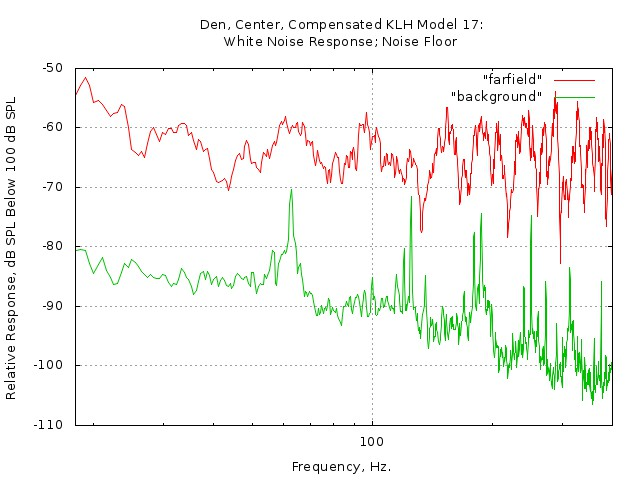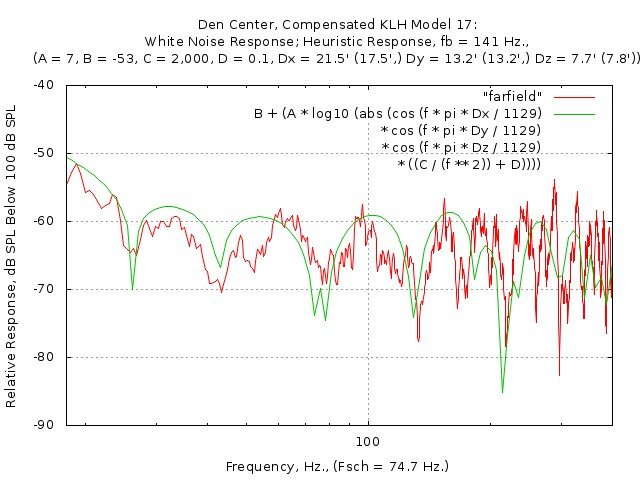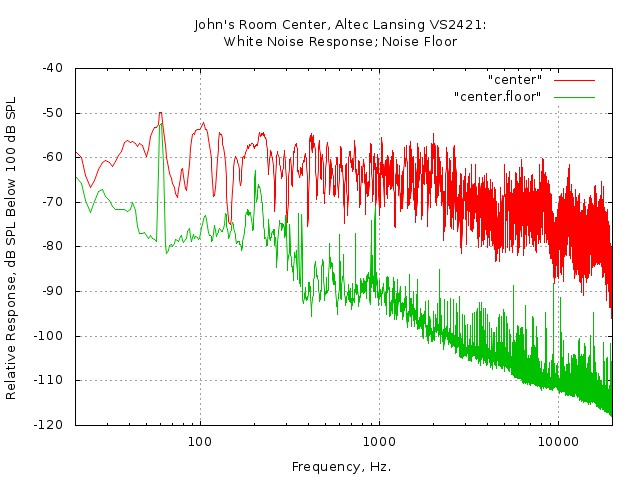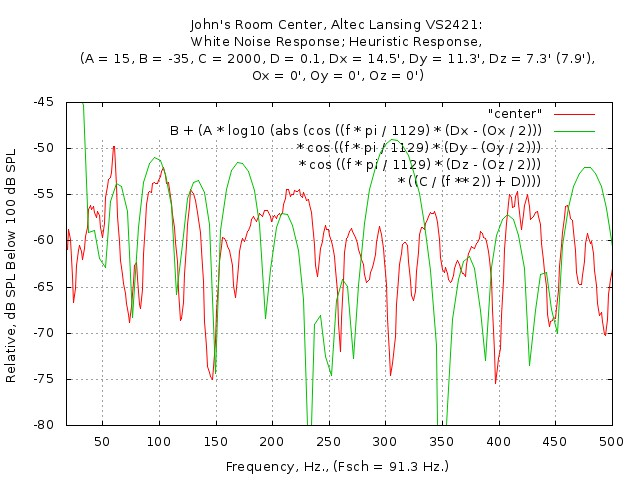john@email.johncon.com http://www.johncon.com/john/

## Room Acoustics

Measurement microphones, (see: Using the Panasonic WM61A as a Measurement Microphone,) are an indispensable tool for measuring room acoustics. Much of this section will reference the outstanding course presentation, "PROJEKTKURS I ADAPTIV SIGNALBEHANDLING: Room Acoustics with associated fundamentals of acoustics," (its in English, and quite formal) and the section will present a heuristic/intuitive development and solution to the same standing wave equations for measuring the frequency response of room acoustics, including two example residential rooms.

## The Heuristic Development:

Consider a listener, listening to a sound wave, `sin (a)`, with a reflective wall behind the listener that reflects the sound with 100% efficiency, normal to the sound wave; from the trigonometric identity for summing two sine waves:

``````

sin (a) + sin (b) =
2 * (cos ((a + b) / 2) *
cos ((a - b) / 2)) .............................(1.1)

``````
```
`a = wt`, frequency, radians per
second

`b = w (t - k)`, frequency, radians
per second, delayed by `k`

```
``````

sin (wt) + sin (w(t - k)) =
2 * cos ((wt + w(t - k)) / 2) *
cos ((wt - w(t - k)) / 2) ......................(1.2)

sin (wt) + sin (w(t - k)) =
2 * cos ((wt + wt - wk) / 2) *
cos ((wt - wt + wk) / 2) .......................(1.3)

sin (wt) + sin (w(t - k)) =
2 * cos ((wt + wt - wk) / 2) *
cos (wk / 2) ...................................(1.4)

sin (wt) + sin (w(t - k)) =
2 * cos (wt - (wk / 2)) * cos (wk / 2) .........(1.5)

sin (wt) + sin (w(t - k)) =
2 * cos (w(t - (k / 2))) * cos (wk / 2) ........(1.6)

``````

Which is Equation 2.63, Page 15, "PROJEKTKURS I ADAPTIV SIGNALBEHANDLING: Room Acoustics with associated fundamentals of acoustics," where:

• `cos (w(t - (k / 2)))` due to exciting function, delayed by ```pi - k / 2```.
• `k = Dx / 1129`, `Dx` the total distance of the reflected wave, both to and from; `1129` is the speed of sound, in feet / second; the distance from the listener to the reflective wall is ```Dx / 2```.
• `w = 2 * pi * f`, conversion of radians per second to frequency.
• `cos (wk / 2) =` standing wave ```= cos (pi * f * Dx / 1129)```, which can be negative, (indicating a phase inversion,) and if measured with a microphone would be ```abs (cos (pi * f * Dx / 1129))```.

Note that for ```cos (pi * f * Dx / 1129) = 0```, (i.e., a null,) ```pi * f * Dx / 1129 = pi / 2```, or:

``````

f = 1129 / (2 * Dx) ................................(1.7)

``````

for the first null; and a null will occur at integer multiples of this frequency, (measured at a distance `Dx / 2` from the reflective wall.) See: Room acoustics for home audio: How to find and treat your room's trouble spots, by Steve Kindig "Problem 1: Standing waves and room resonance modes," which has the same equation.

Further, if another reflective wall is placed in front of the listener, (that reflects the sound with 100% efficiency, normal to the sound wave, i.e., there is no dissipation of energy,) at a distance of `Dx` from the reflective wall in back of the listener, (i.e., the listener is half way between the two parallel reflective walls, the reflective walls being separated by a distance of `Dx`,) the situation is not altered, and is identical to the Room acoustics for home audio: How to find and treat your room's trouble spots page.

As a heuristic, if another set of parallel reflective walls, perpendicular to the first set of reflective walls, `cos (w(t - (k / 2)))` would be the exciting function, and another standing wave scenario would exist, ```cos (pi * f * Dy / 1129)```, for a composite of ```cos (pi * f * Dx / 1129) * cos (pi * f Dy / 1129)```, and ```cos (pi * f * Dx / 1129) * cos (pi * f * Dy / 1129) * cos (pi f * Dz / 1129)``` for three sets of walls-just as an intuitive/heuristic development of Equation 2.68, Page 16, "PROJEKTKURS I ADAPTIV SIGNALBEHANDLING: Room Acoustics with associated fundamentals of acoustics".

But note that the range of applicability is below the Schroeder cutoff frequency, `Fsch`.

From Equation 3.28, Page, 28, "PROJEKTKURS I ADAPTIV SIGNALBEHANDLING: Room Acoustics with associated fundamentals of acoustics":

``````

Fsch = 1900 * sqrt (T / V)

``````

where, from Equation 3.11, Page 21, "PROJEKTKURS I ADAPTIV SIGNALBEHANDLING: Room Acoustics with associated fundamentals of acoustics":

``````

T = 0.161 V / A

``````

and substituting:

``````

Fsch = 1900 * sqrt (0.161 / A) .....................(1.8)

``````

where `A` is the area of the surfaces in the room, (square meters,) i.e., the sum of the area of all six walls.

Note that the derivation, above, assumed walls with no energy loss, at any frequency, (not to mention that sound intensity decreases with the square of the distance, `Dx`, for the reflected sound, which was ignored, too,) which will be accommodated in the next section.

## Empirical Verification:

Two residential rooms will be analyzed, one of reasonable physical dimensions, (a den, with a typical HiFi sound system,) and another with nearly cubical physical dimensions, (a bedroom converted to an office with a near-field sound system.)

The den has wallboard walls, brick fireplace, and wood floors, in addition to furniture-all of which absorb sound. Both wallboard and wood floors do not absorb sound at very low frequencies, but do, (by about ```20 dB```,) at higher frequencies, i.e., an integration process, (i.e., a pole at ```0 f``` in the transfer function,) and a zero that runs, typically, between `100` and `200` Hz., canceling the pole. The zero in the transfer function used in the figure is when `D = 0.1 = C / f^2 = 2000 / f^2`, or `fb`, the break point for the zero, is `141` Hz.### Figure I. Den Center Frequency Response

Figure I is a plot of the frequency response of the den, measured in the center of the room, and the room noise floor. White noise was used as the signal source for the frequency response.

Since a signal source with a flat frequency response was required, an acoustic suspension speaker was chosen, (KLH Model 17,) since the low frequency roll off response of closed box speakers is gentle and predictable. The speaker amplifier was driven by the whiteLF_sound(1) program from the sine_sound.tar.gz archive to linearize the response of the KLH speaker at low frequencies with the following STDIN commands:

```
p 5.3
g 0.006
a 20.0

```

which provided a flat white noise sound signal, (approximately 40 dB gain at 5.3 Hz., over the flat band high frequency gain,) from the speaker for the analysis of the frequency response of the den. A measurement microphone and amplifier, as described in the Using the Panasonic WM61A as a Measurement Microphone page, was placed in the exact center of the room, and a Linux PC running the Baudline FFT time-frequency browser designed for scientific visualization of the spectral domain used to analyze and record the sound pressure level, (SPL,) signal at the room's center.### Figure II. Den Center Frequency Response and Heuristic Room Response

Figure II is a plot of the frequency response of the den, overlayed with the heuristic/intuitive response of an empty room of similar dimensions and characteristics. The Schroeder cutoff frequency of the den is 74.7 Hz., (which is, approximately, the limit of the heuristic analysis' accuracy.)

The office has wallboard walls and wood floors, in addition to office furniture, (computer tables, printer stands, bookshelfs, etc.,) all of which absorb sound. Both wallboard and wood floors do not absorb sound at very low frequencies, but do, (by about `20 dB`,) at higher frequencies, i.e., an integration process, (i.e., a pole at `0 f` in the transfer function,) and a zero that runs, typically, between `100` and `200` Hz., canceling the pole. The zero in the transfer function used in the figure is when `D = 0.1 = C / f^2 = 2000 / f^2`, or `fb`, the break point for the zero, is `141` Hz. The listening postion, (a near-field configuration of computer speakers, Altec-Lansing, Model VS2421,) is offset. The `cos (pi * f * Dx / 1129)` then becomes ```cos ((pi * f * Dx / 1129) - (pi * f * Ox / 1129)) = cos ((pi * f / 1129) * (Dx - (Ox / 2))```, where `Ox` is the offset in the `x` direction, (noting that when `Ox = Dx / 2`, the listening position is at the `x` axis wall.)### Figure III. Office Center Frequency Response

Figure III is a plot of the frequency response of the office, measured in the center of the room, (the speaker frequency response was not compensated,) and the room noise floor. White noise was used as the signal source for the frequency response.### Figure IV. Office Center Frequency Response and Heuristic Room Response

Figure IV is a plot of the frequency response of the office center, (the speaker frequency response was not compensated,) overlayed with the heuristic/intuitive response of an empty room of similar dimensions and characteristics. The Schroeder cutoff frequency of the den is 91.3 Hz., (which is, approximately, the limit of the heuristic analysis' accuracy.)

There is a common misconception that the room response can be equalized. To demonstrate the fallacy:### Figure V. Office Listener Position Frequency Response Overlayed with Moving the Microphone Two Inches

Figure V is a plot of the frequency response of the office at the listener's position, (about 2 feet in both the `x` and `y` directions from the center,) and then moving the microphone 2 inches in the `y` direction, and retaking the frequency response data.

Note that:

• There is a significant change in the frequency response between the center of the room, and the listener's position, (a distance change of a couple of feet,) and;
• There is a significant change in the frequency response by moving the microphone, (or the listener's ears,) a couple of inches.

## Addendum, Acoustic Measurments with White Noise

### Square Wave Calibration Signal:

Suppose that a sound system is calibrated such that a 0 dBFS square wave signal, (i.e., peak readings of the square wave signal are +32767 and -32768 for 16 bit data, where `2^16 = 65536`,) then the RMS, Root Mean Square, of the square wave signal would be 32767.5, corresponding to the maximum output square wave signal level. This would correspond, through gain calibration, to 100 dB SPL, consistent with OSHA 1910.95, EBU R68-2000, and, EBU R89-1997 compliance.

A 0 dBFS square wave calibration signal produces the maximum power output of the sound system.

For 85 dB SPL of the maximum power output of the sound system, (i.e., 100 dB SPL for a square wave,) the signal must be reduced by a factor of 15 dB, and, ```10^(15 / 20) = pow (10, 15 / 20) = 5.62341325190349080395```, or the signal should be multiplied by a factor of ```1 / 5.62341325190349080395 = 0.17782794100389228012```.

Inexpensive sound level meters low pass filter the rectified signal received to produce the mean of the signal, Vmean, which is the same as the RMS, Root Mean Square, value of the signal, `Vrms` for the square wave.

The actual average rectified sound level meter reading is correct, (but may be multiplied by a conversion factor of `1.11072073453959156175` if the meter is calibrated to present values for sine wave RMS, Root Mean Square.)

### Sine Wave Calibration Signal:

Suppose that a sound system is calibrated such that a 0 dBFS sine wave signal, (i.e., peak readings of the sine wave signal are +32767 and -32768 for 16 bit data, where `2^16 = 65536`,) then the RMS, Root Mean Square, of the sine wave signal would be ```32767.5 / sqrt (2) = 23170.12145253019600583234```, corresponding to the maximum output sine wave signal level. A 0 dBFS sine wave calibration signal produces ```1 / sqrt (2) = 0.70710678118654752440 = -3.01029995663981195215``` dBFS of the maximum power output of the sound system, (which is the defacto reference "standard" of the industry.) For 85 dB SPL of the maximum power output of the sound system, (i.e., 100 dB SPL for a square wave,) the signal must be reduced by a factor of ```15 - 3.01029995663981195215 = 11.98970004336018804785 dB```, and, ```10^(11.98970004336018804785 / 20) = pow (10, 11.98970004336018804785 / 20) = 3.97635364383525332586```, or the signal should be multiplied by a factor of ```1 / 3.97635364383525332586 = 0.25148668593658708166```.

Inexpensive sound level meters low pass filter the rectified signal received to produce the mean of the signal, Vmean, and then multiply this value by a conversion factor to obtain the RMS, Root Mean Square, value of the signal, `Vrms`, from Full-wave rectification:

``````

Vmean = 2 * Vpeak / pi
Vpeak = sqrt (2) * Vrms

Vmean = 2 * (sqrt (2) * Vrms) / pi
Vrms = Vmean * (pi / (2 * sqrt (2)))
= Vmean * 1.11072073453959156175

``````

which is the value of the conversion factor for a low pass filtered rectified sine wave calibrated sound level meter.

### Uniformly Distributed White Noise Calibration Signal:

Suppose that a sound system is calibrated such that a 0 dBFS uniformly distributed white noise signal, (i.e., peak readings of the uniformly distributed white noise signal are +32767 and -32768 for 16 bit data, where ```2^16 = 65536```,) then the RMS, Root Mean Square, of the noise signal would be, from Uniform distribution (continuous):

The mean of a uniform distribution, (i.e., white noise,) bounded on the interval a to b, with a probability density function of `1 / (b - a)` is:

``````

mean = (a + b) / 2

``````

And, the variance around the mean:

``````

variance = (1 / 12) * ((b - a)^2)

``````

where the standard deviation around the mean is:

``````

standard deviation = sqrt (variance)

``````

which is the RMS, Root Mean Square, of the noise signal, and, substituting:

``````

Vrms = sqrt ((1 / 12) * ((32767 - (-32768))^2))
= 18918.32494567106223855353

``````

A 0 dBFS uniformly distributed white noise calibration signal produces ```18918.32494567106223855353 / 32767.5 = 0.57735026918962576451 = -4.77121254719662437297``` dBFS of the maximum power output of the sound system.

For 85 dB SPL of the maximum power output of the sound system, (i.e., 100 dB SPL for a square wave,) the signal must be reduced by a factor of ```15 - 4.77121254719662437297 = 10.22878745280337562703``` dB, and, ```10^(10.22878745280337562703 / 20) = pow (10, 10.22878745280337562703 / 20) = 3.24667915475098921473```, or the signal should be multiplied by a factor of ```1 / 3.24667915475098921473 = 0.30800702882410229172```.

Inexpensive sound level meters low pass filter the rectified signal received to produce the mean of the signal, `Vmean`:

``````

mean = (0 + 32767.5) / 2 = 16383.75

variance = (1 / 12) * ((32767.5 - 0)^2)
= 89475754.6875

``````

which is the variance of the elements around the mean of 16383.75, or:

``````

standard deviation = sqrt ((1 / 12) * ((32767.5 - 0)^2))
= 9459.16247283553111927677

``````

which is the standard deviation of the elements around the mean of 16383.75. Adding the two values together, Root Mean Square, to obtain the RMS, Root Mean Square, value of the signal, `Vrms`:

``````

Vrms = sqrt ((16383.75^2) + (9459.16247283553111927677^2))
= 18918.32494567106223855353

``````

The actual average rectified sound level meter reading is correct, (but may be multiplied by a conversion factor of 1.11072073453959156175 if the meter is calibrated to present values for sine wave RMS, Root Mean Square.)

### Notes, Acoustic Measurments with White Noise

 EBU R68-2000 states that:

... an audio signal level can be defined in terms of an alignment signal that is a sine wave signal which has a level (the alignment level) which is 9 dB ... below the permitted maximum level of the audio programme (the terms "alignment level" and "permitted maximum" are defined in ITU-R Recommendation BS.645 [which concerns test signals and metering to be used on international sound programme connections] ...

 EBU R89-1997 states that:

... [The] alignment Level should be 9 dB below PML, that is -12 dBFS ...

Recordings should be made such that the Permitted Maximum Level, PML, is -3 dBFS. (dB Full Scale) ...

Alignment Level should be 9 dB below PML, that is -12 dBFS ...

... [The] maximum coding level (0 dBFS) Audio alignment level ... [should be E000 for negative peaks, 1FFF for positive peaks, with 0 dB FS 8000 for negative peaks, and 7FFF for positive peaks] ...

 Although its rather convoluted, it appears that the level on a CD should be -18 dBFS, where 0 dBFS is the peak value of a sine wave, (EBU R68-2000,) and -15 dBFS, where 0 dBFS is the RMS value of a sine wave with a peak value of 0 dBFS, (EBU R89-1997.) Note the confusion: is the program level -15 dB below 0 dBFS, or -18 dB below dBFS?

The industry's common "standard" for the maximum power output is a sine wave with instantaneous peaks of 0 dBFS. However, using a standard of a square wave of 0 dBFS is not ambiguous-which is what was used in this derivation.

A license is hereby granted to reproduce this design for personal, non-commercial use.

THIS DESIGN IS PROVIDED "AS IS". THE AUTHOR PROVIDES NO WARRANTIES WHATSOEVER, EXPRESSED OR IMPLIED, INCLUDING WARRANTIES OF MERCHANTABILITY, TITLE, OR FITNESS FOR ANY PARTICULAR PURPOSE. THE AUTHOR DOES NOT WARRANT THAT USE OF THIS DESIGN DOES NOT INFRINGE THE INTELLECTUAL PROPERTY RIGHTS OF ANY THIRD PARTY IN ANY COUNTRY.

So there.

john@email.johncon.com

http://www.johncon.com/john/
http://www.johncon.com/ntropix/
http://www.johncon.com/ndustrix/
http://www.johncon.com/nformatix/
http://www.johncon.com/ndex/